Custom SearchPRACTICE PROBLEMS: Give the equation; the length of a; and the length of the focal chord for the parabola, which is the locus of all points equidistant from the point and the line, given in the following problems: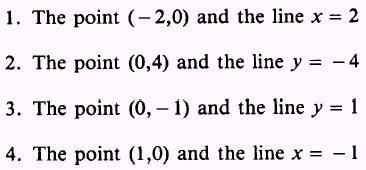ANSWERS: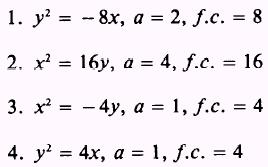Up to now, all of the parabolas we have dealt with have had a vertex at the origin and a corresponding equation in one of the four following forms: 1. y2 = 4ax 2. y2 = - 4ax 3. x2 = 4ay 4. x2 = - 4ay We will now present four more forms of the equation of a parabola. Each one is a standardized parabola with its vertex at point V(h,k). When the vertex is moved from the origin to the point V(h,k), the x and y terms of the equation are replaced by (x - h) and (y - k). Then the standard equation for the parabola that opens to the right (fig. 2-9, view A) is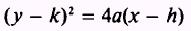The four standard forms of the equations for parabolas with vertices at the point V(h,k) are as follows:The method for reducing an equation to one of these standard forms is similar to the method used for reducing the equation of a circle. EXAMPLE: Reduce the equation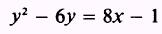to standard form. SOLUTION: Rearrange the equation so that the second-degree term and any first-degree terms of the same unknown are on the left side. Then group the unknown term appearing only in the first degree and all constants on the right: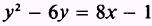Then complete the square in y: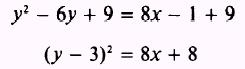To get the equation in the form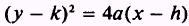factor an 8 out of the right side. Thus,is the equation of the parabola with its vertex at (-1,3). PRACTICE PROBLEMS: Reduce the equations given in the following problems to standard form: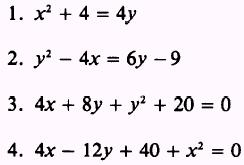ANSWERS: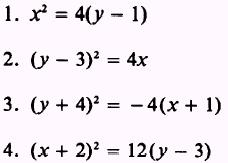Integrated Publishing, Inc. - A (SDVOSB) Service Disabled Veteran Owned Small Business